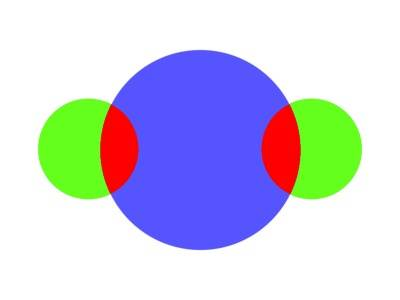# Would Newton's shell theorem prevent binary planet systems?

• I
Would the shell theorem prevent a binary planet system, with two ideally equal masses, structure etc?

mfb
Mentor
The shell theorem is true, and there are probably binary planets around (and Pluto/Charon are similar to a binary planet). Clearly not.

Why would you expect that?

The shell theorem is true, and there are probably binary planets around (and Pluto/Charon are similar to a binary planet). Clearly not.

Why would you expect that?
I meant two planets of the same mass and make up(ideally).take these two binary planets, of equal construction(green+red). In the shell theorem, the green part of the planet, would have no gravitational pull on the red part of the planet, so there is nothing to hold the red part on...and so on.

#### Attachments

Orodruin
Staff Emeritus
Homework Helper
Gold Member
2021 Award
The shell theorem holds for a spherically symmetric distribution. Your setup is not spherically symmetric.

The shell theorem holds for a spherically symmetric distribution. Your setup is not spherically symmetric.
yes, I think you're correct....I've jumped the gun again...The shell theorem holds for a spherically symmetric distribution. Your setup is not spherically symmetric.
or maybe I'm right(probably not....)...surely if the shell theorem holds for a whole sphere, it would also hold for a symmetrical two body system...?

yes,if I'm right; in the process of the formation of a binary planet system, from dust, one of the planets, would have to be smaller than the other, by some factor, I would guess.

Ken G
Gold Member
You are confusing the term "symmetric" (by which you mean a mirror symmetry) with "spherically symmetric" (which requires dependence on nothing but distance from a center point, no angular dependence). The latter is much more restrictive, and is the only time the shell theorem applies.

You are confusing the term "symmetric" (by which you mean a mirror symmetry) with "spherically symmetric" (which requires dependence on nothing but distance from a center point, no angular dependence). The latter is much more restrictive, and is the only time the shell theorem applies.
ok so I may well have jumped the gun, but is there still something to the idea?

So maybe the shell theorem wouldn't apply exactly, but just enough to cause a binary system, to need to have two different sized planets?

ok, there's probably next to nothing in the idea...

mfb
Mentor
It doesn't apply at all to the whole system, not even approximately. You can use it for the planets separately. For a test mass on the surface of a planet, you can calculate the gravitational force from that planet and the force from the other planet with it. The former will win by a large margin.

or maybe I'm right(probably not....)...surely if the shell theorem holds for a whole sphere, it would also hold for a symmetrical two body system...?
Your saying that because the second planet is outside the first planet, according to the shell theorem, the second planet exerts no gravitational force on the first planet, right? But in the shell theorem, the mass outside the shell is counter-balanced by the mass of the shell on the opposite side - it's spherically symmetric. In the two planet example, there's no mass on the other side of the first planet to counter-balance the gravitational attraction of the second planet.